﻿ Free 5th Grade Math Worksheets PDF

Home / Math Worksheets / 5th Grade Math Worksheets

# Free 5th Grade Math Worksheets PDF

Download free printable 5th Grade math worksheets with answer key in PDF below. Our 5th Grade math worksheets can be used in the classroom or for homeschooling. These math worksheets for 5th Grade contain also the answer key and a special link to the online game which helps students to improve 5th Grade math skills.

You can also play Math Games for Grade 5 online here →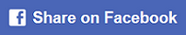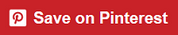## 5th Grade Math Worksheets Printable

### Multiplication table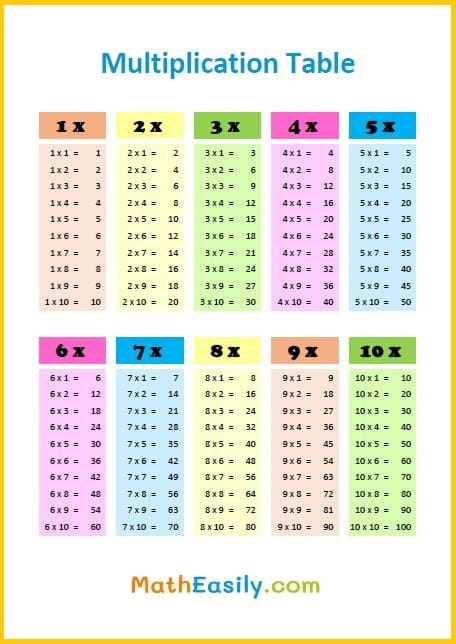### School timetable

School timetable template in PDF and EXCEL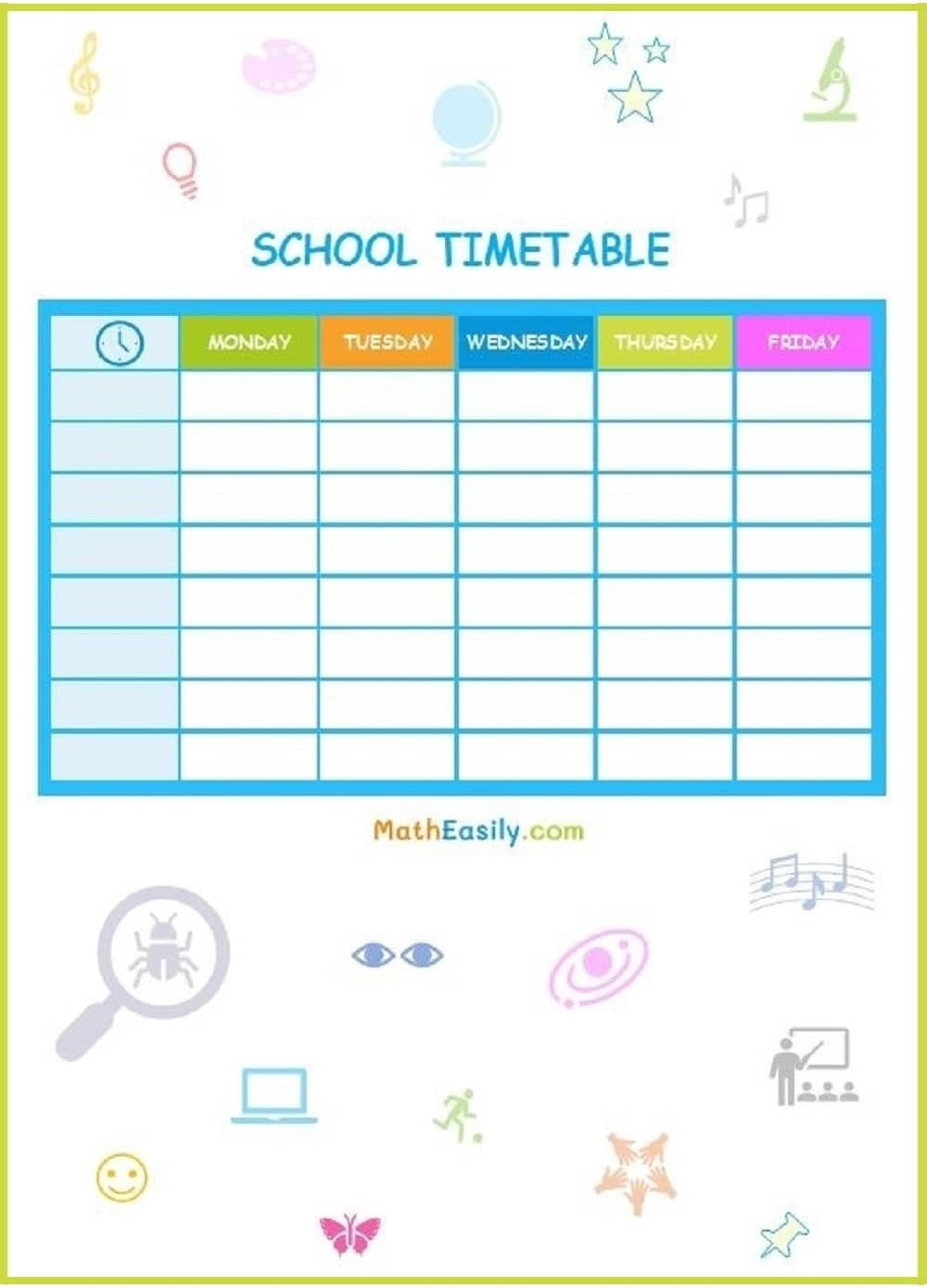### Subtraction pyramids

Fill in subtraction pyramid puzzles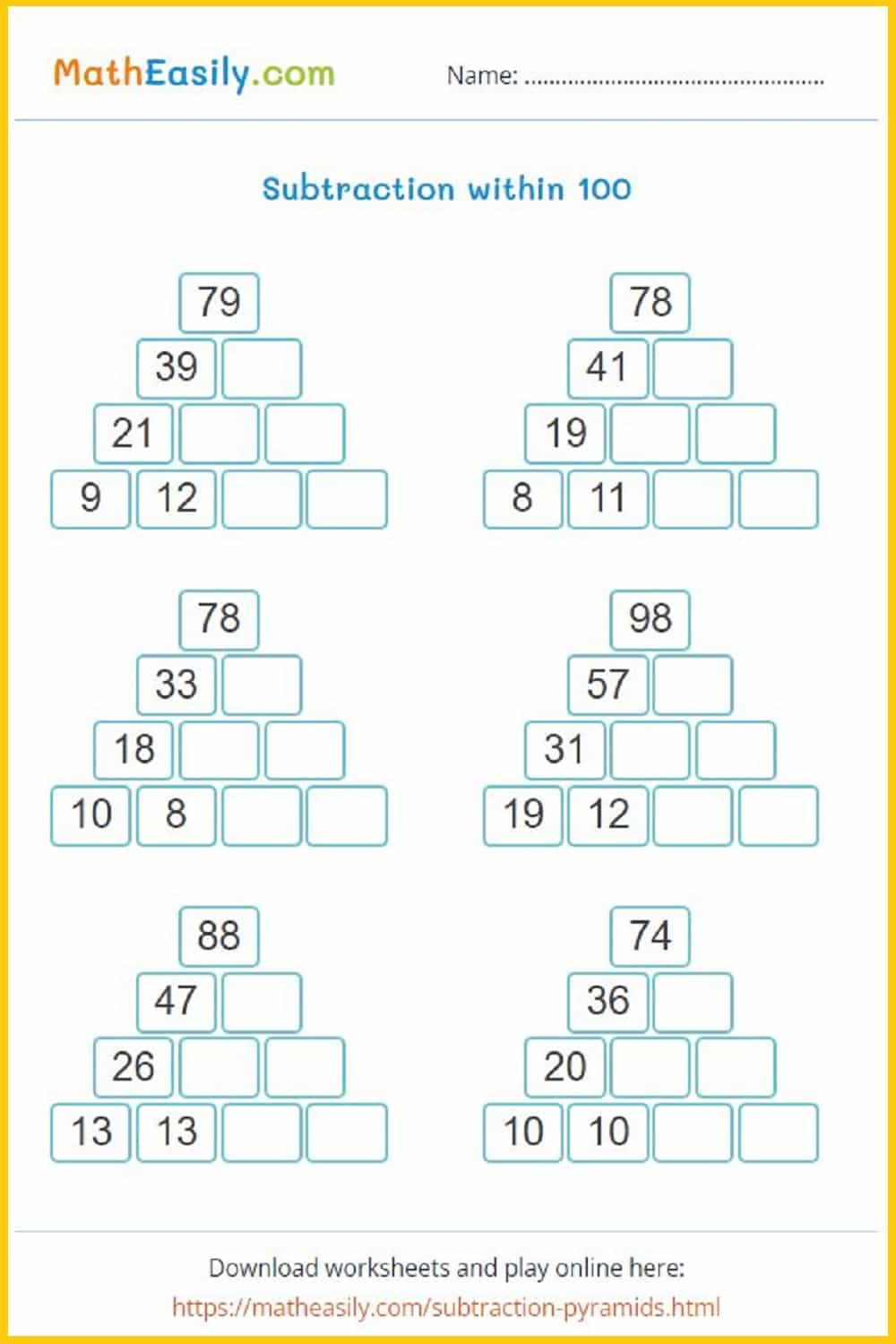### Maths puzzles

Mixed addition and subtraction puzzles up to 100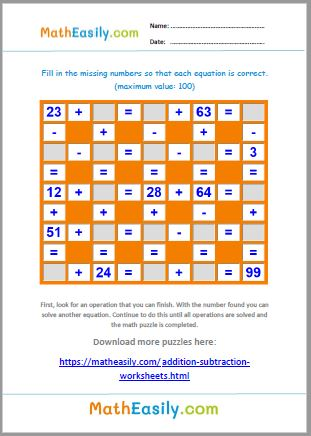### 100 Maths puzzles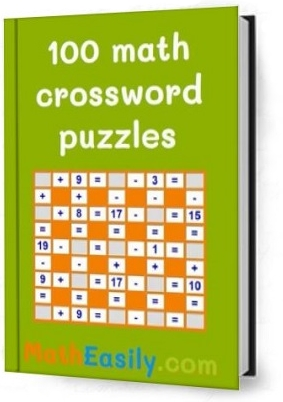Look inside here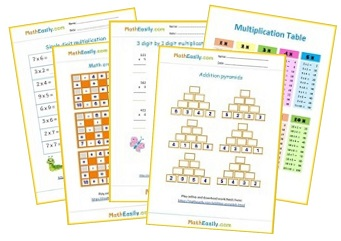Free Math Worksheets

## Multiplication Worksheets Grade 5 PDF

Our free multiplication worksheets for Grade 5 (PDF) help kids to practice a certain task. These printable 5th Grade multiplication worksheets are prepared with the aim of to help students learn multiplication in a fun way.

### Multiplication board game

Learning multiplication is one of the most challenging tasks in math. To help our kids to learn it easily I designed this printable multiplication board game in PDF.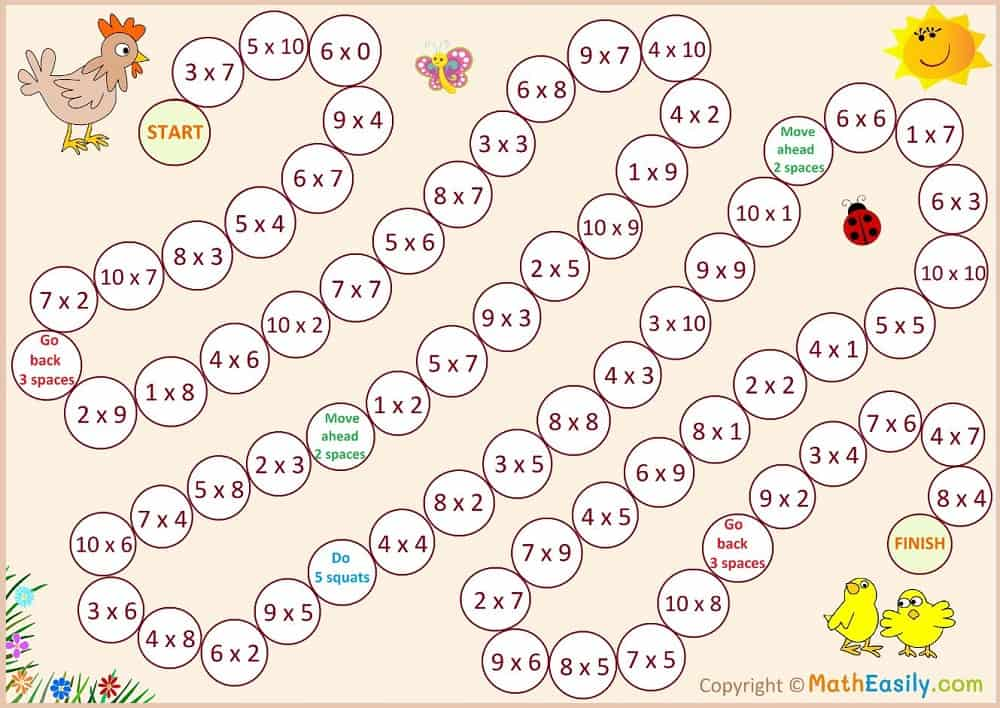### Single digit multiplication

Practice single digit multiplication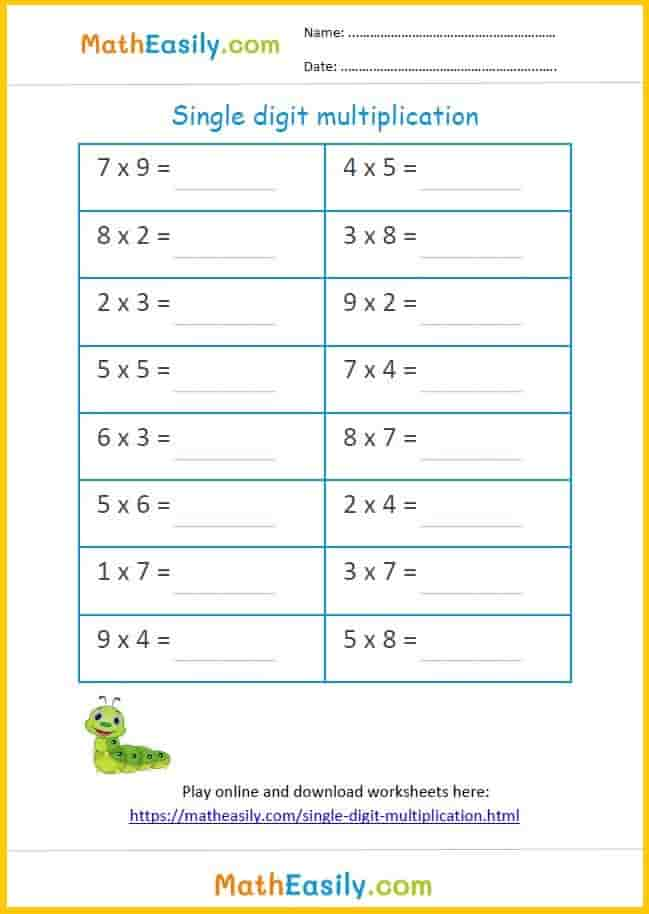### Single digit multiplication

Practice single digit multiplication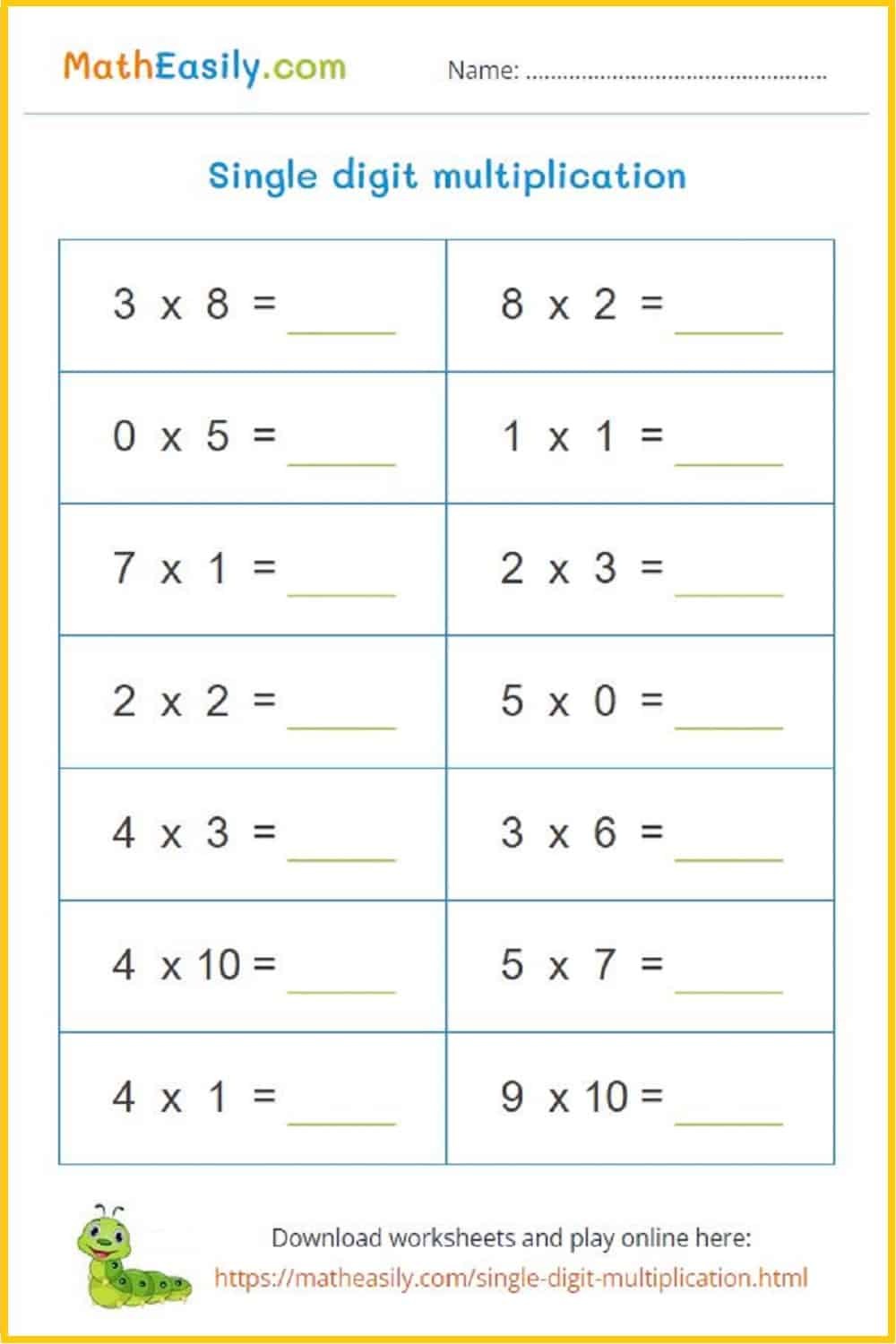### Multiplication pyramids

Fill in multiplication pyramid puzzle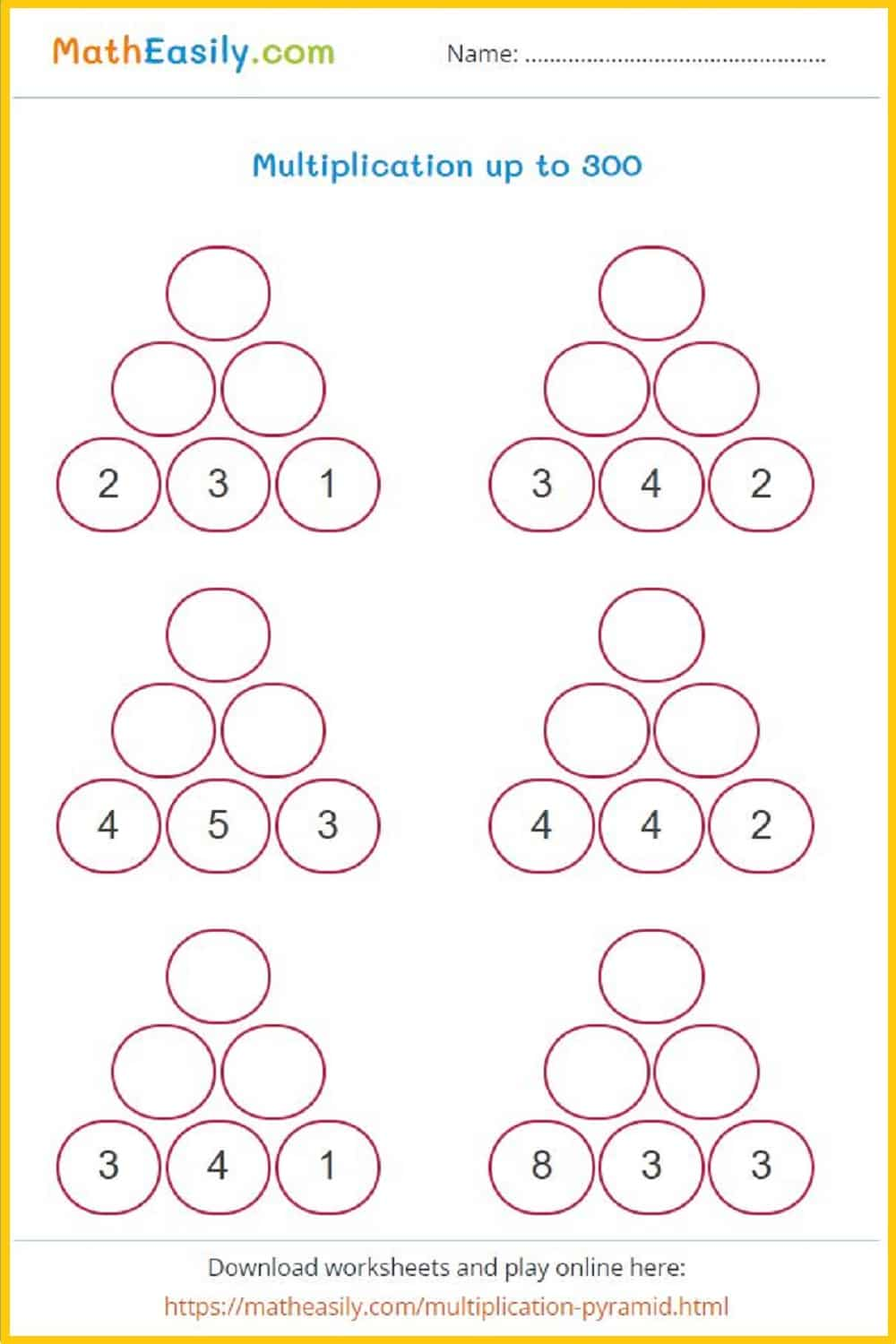### Multiplication pyramids

Fill in multiplication pyramid puzzle### Multiplication comparison

Compare results of the multicplication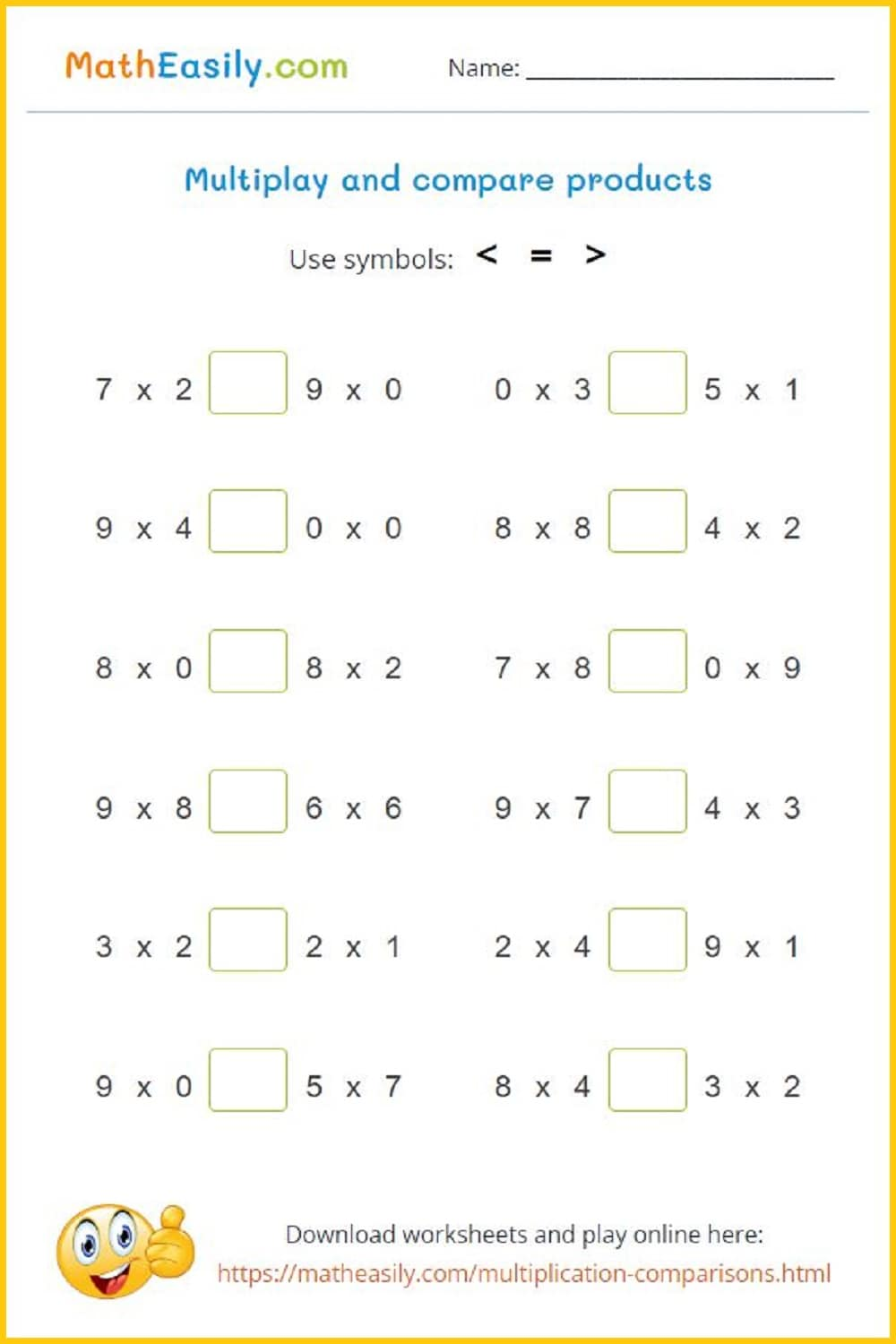### Multiplication comparison

Compare results of the multicplication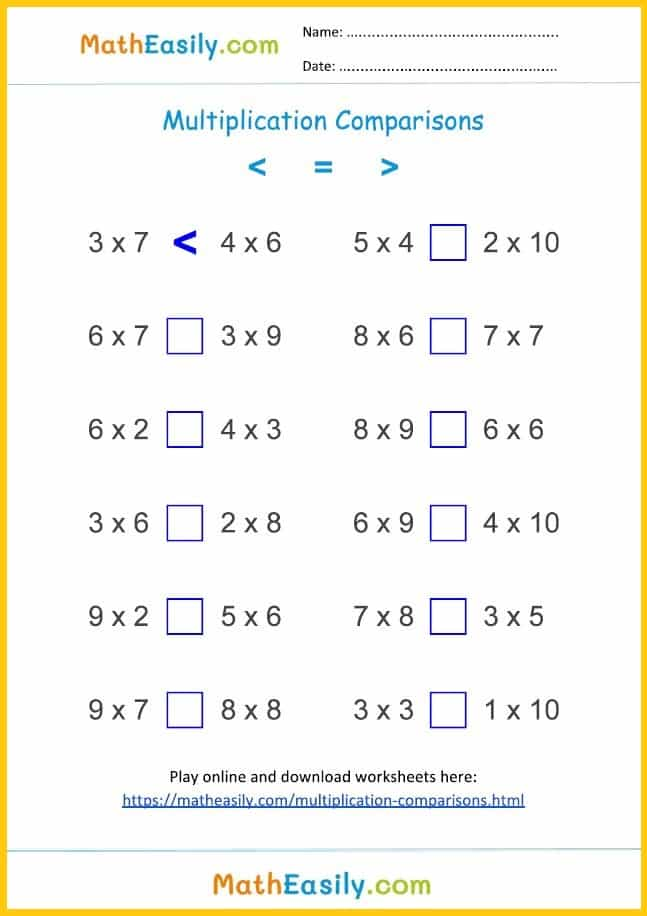### 2 digit by 1 digit

Multiply 2 digit by 1 digit numbers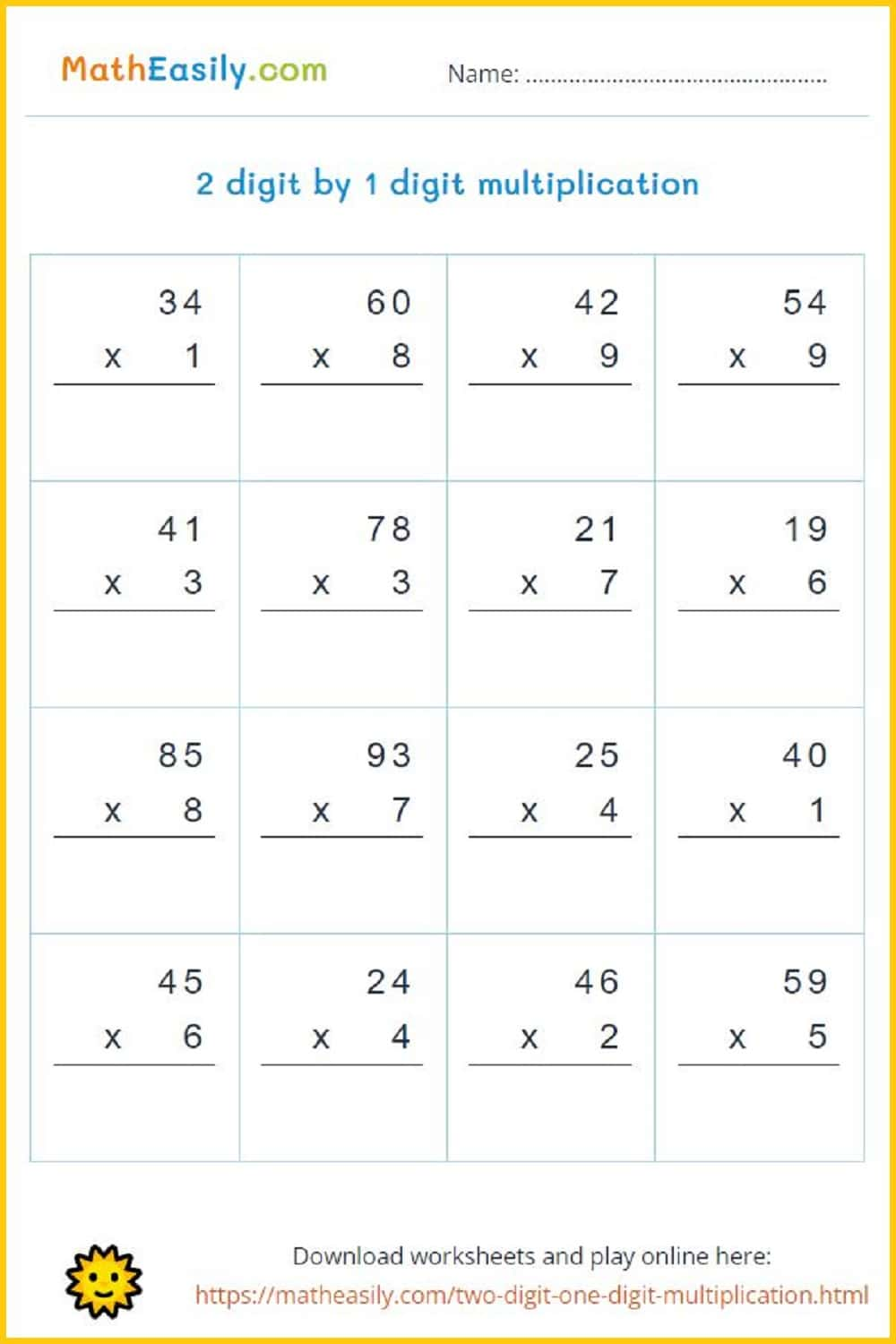### 2 digit by 1 digit

Multiply 2 digit by 1 digit numbers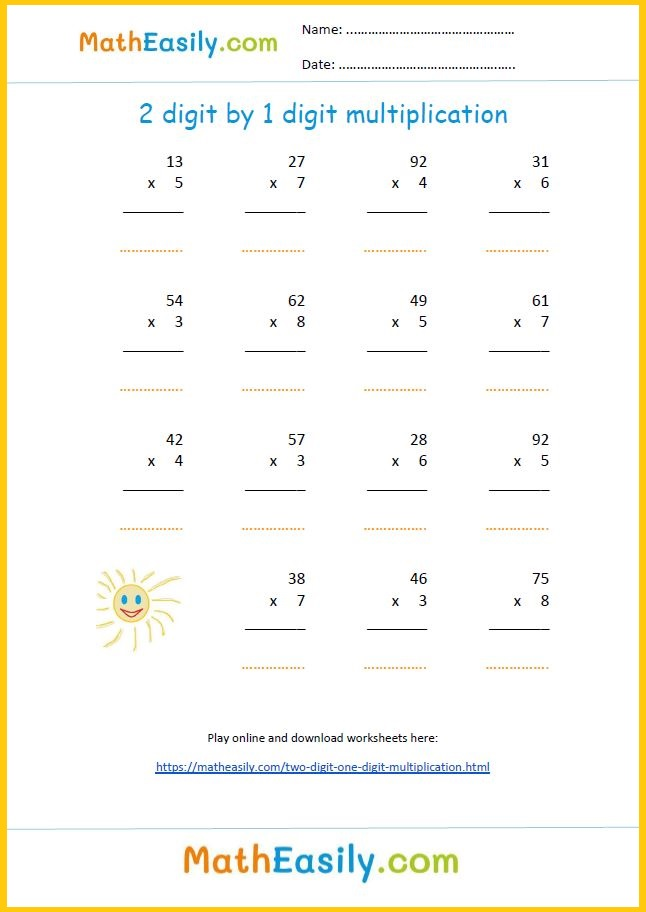### 3 digit by 1 digit

Multiply 3 digit by 1 digit numbers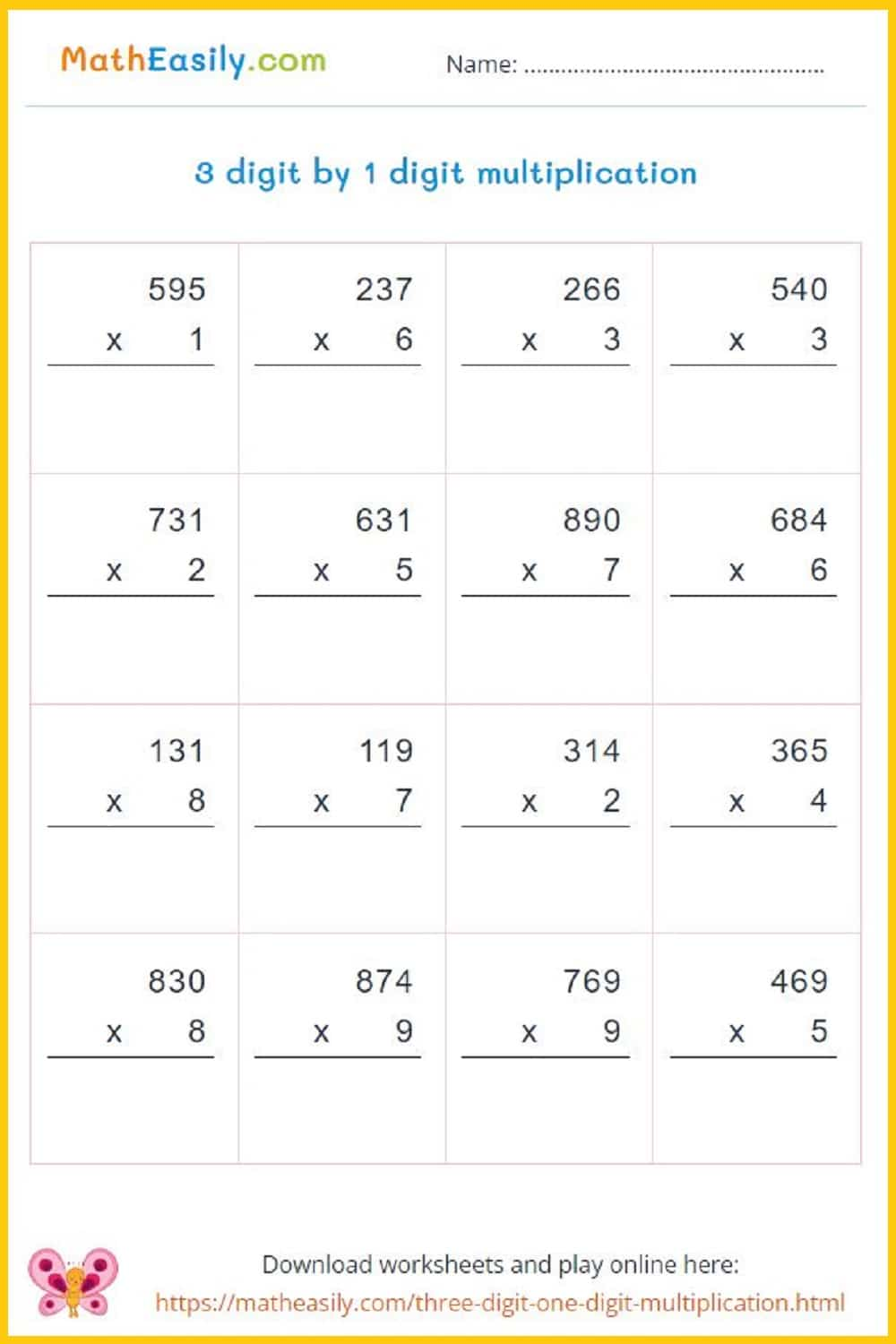### 3 digit by 1 digit

Multiply 3 digit by 1 digit numbers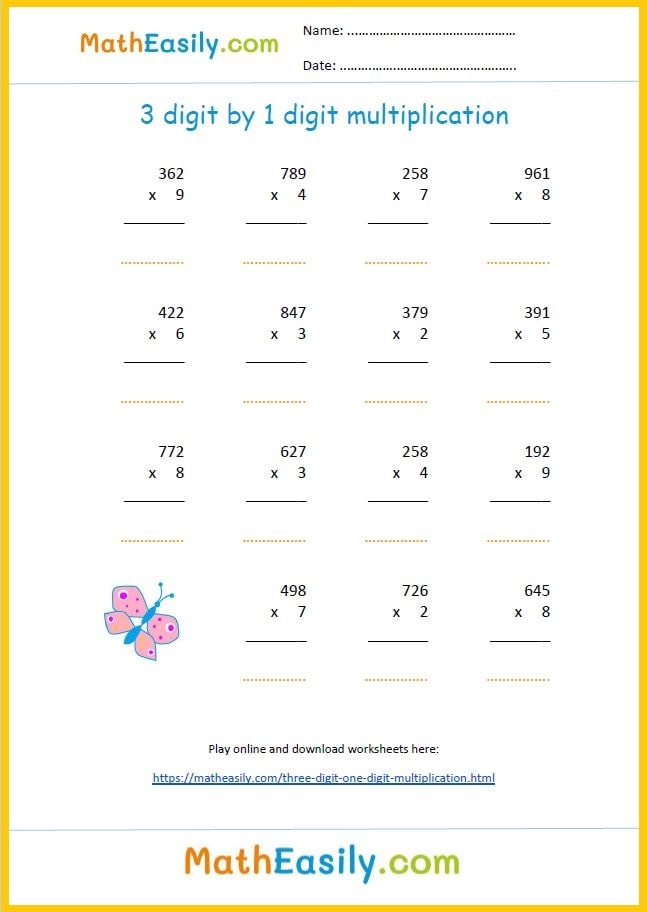### 2 digit multiplication

Multiply 2 digit by 2 digit numbers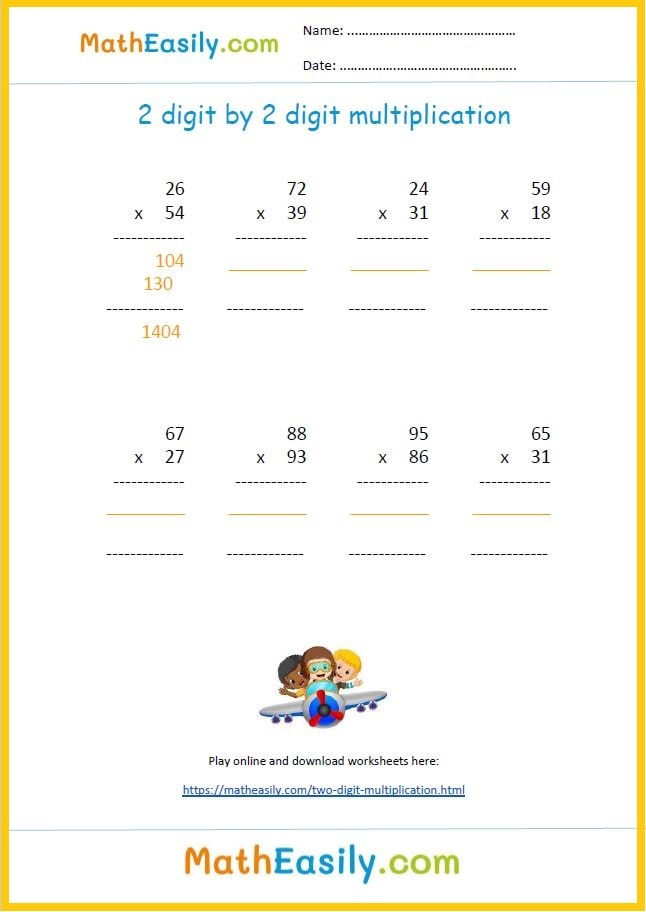### 2 digit by 2 digit

Multiply 2 digit by 2 digit numbers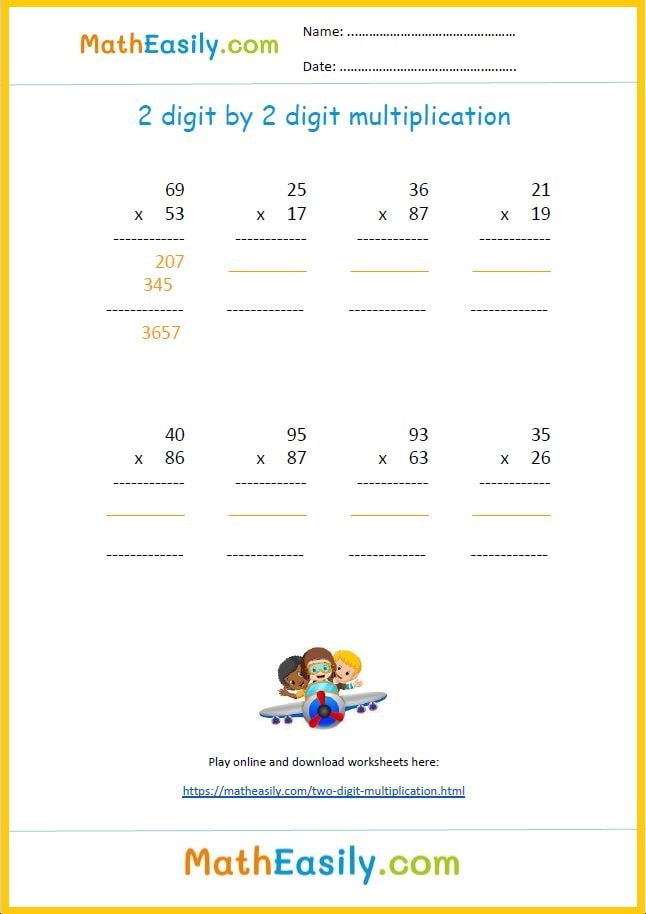### 2 digit by 2 digit

Multiply 2 digit by 2 digit numbers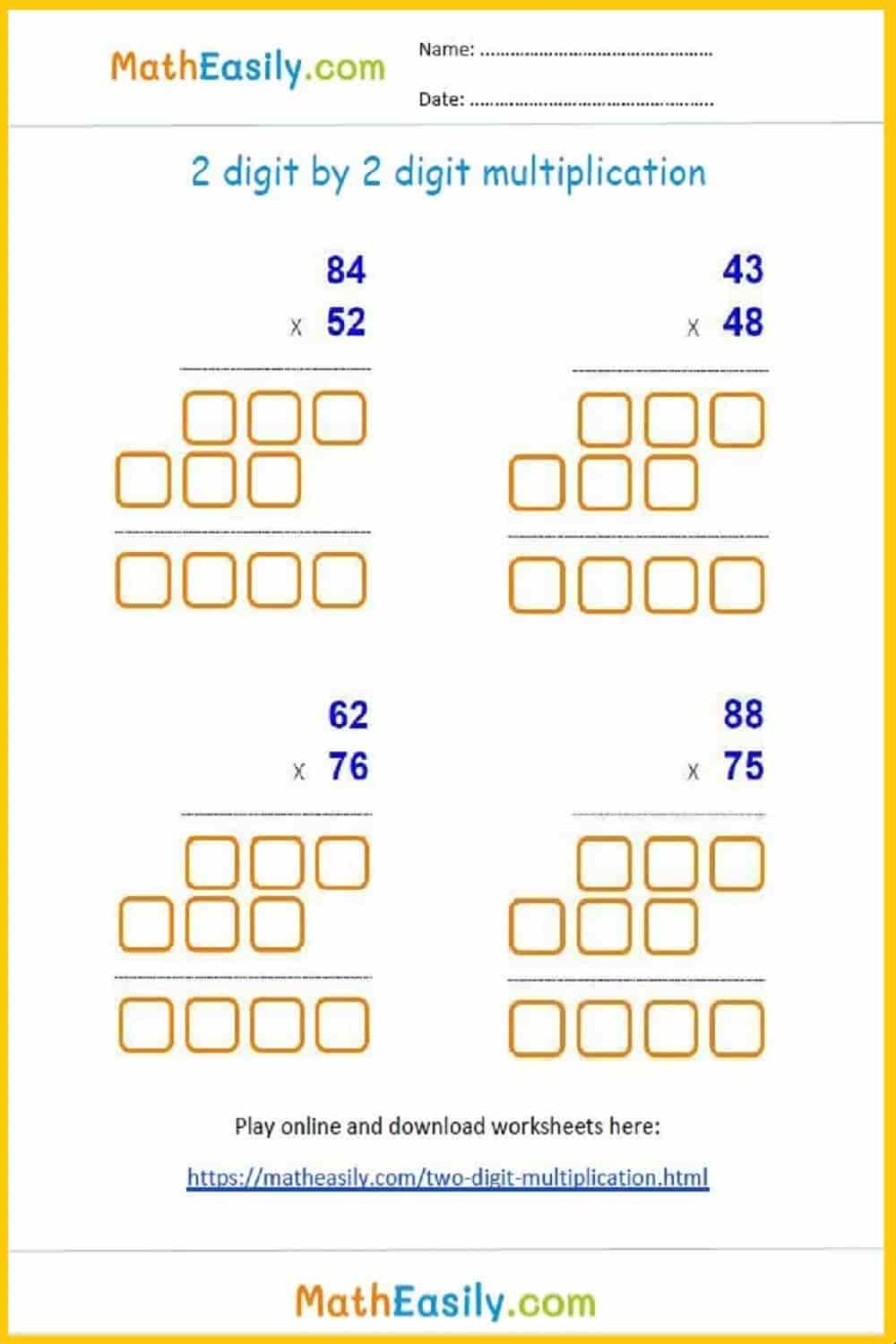### 2 digit by 2 digit

Multiply 2 digit by 2 digit numbers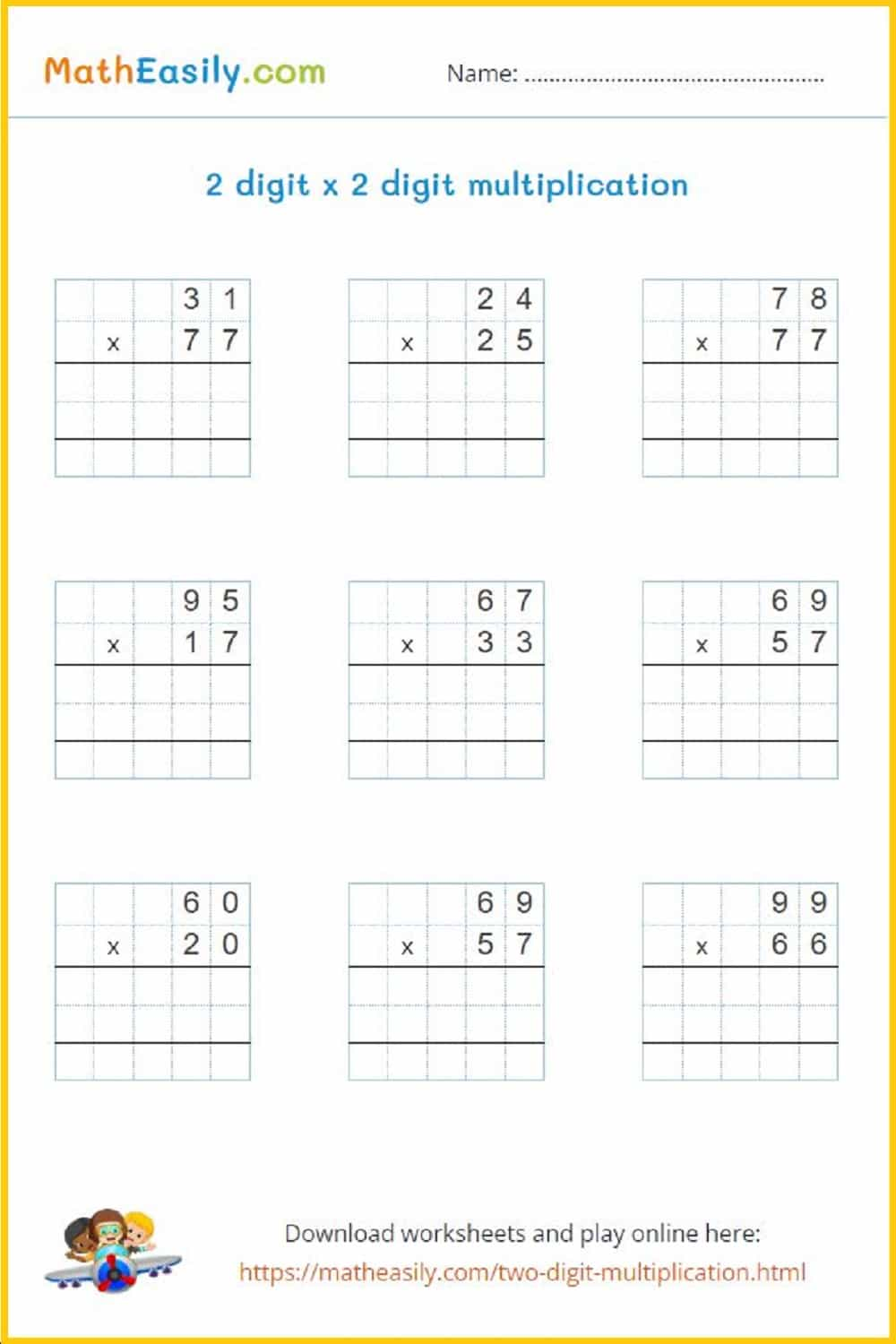### 3 digit by 2 digit

Multiply 3 digit by 2 digit numbers### 3 digit by 2 digit

Multiply 3 digit by 2 digit numbers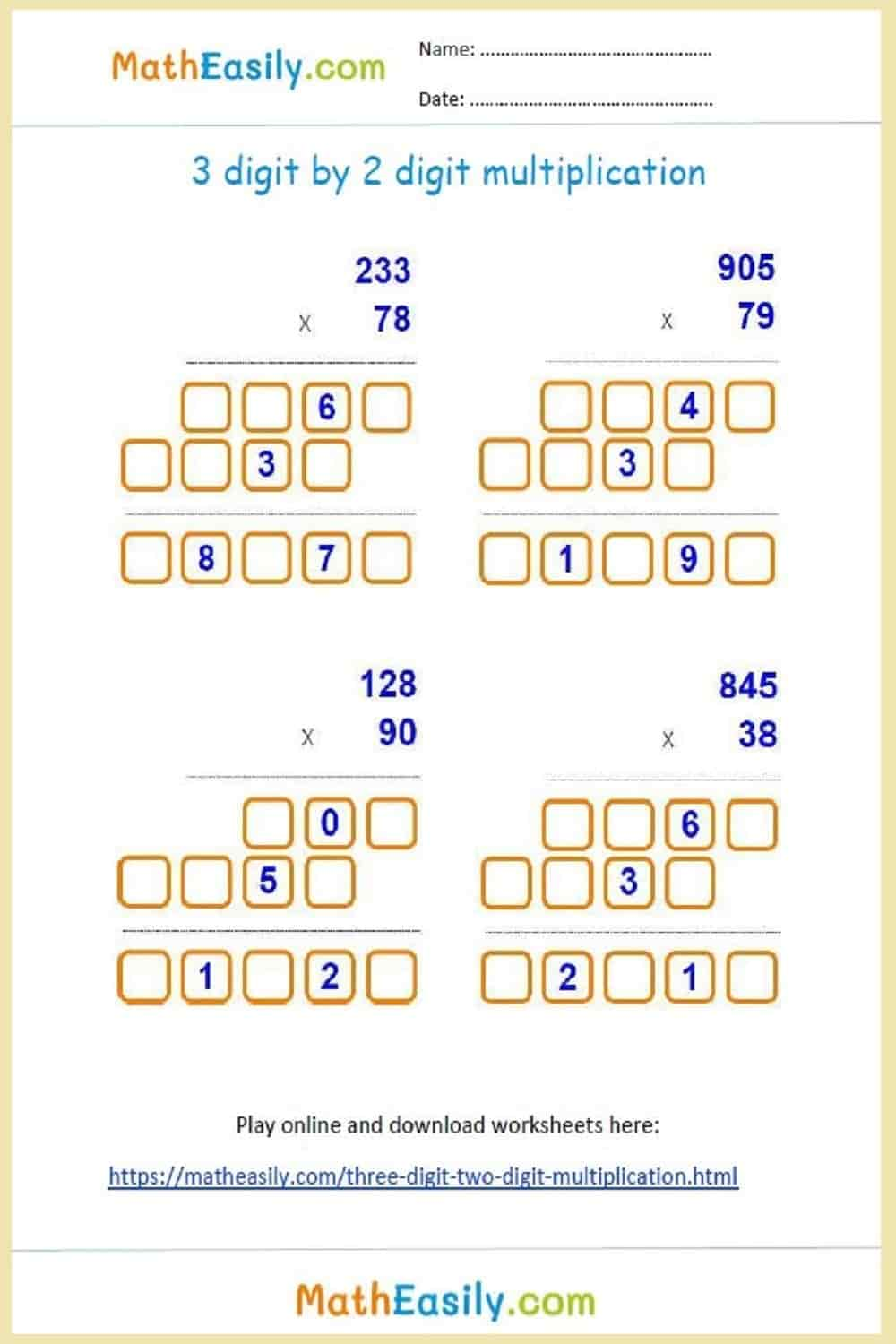### 3 digit by 2 digit

Multiply 3 digit by 2 digit numbers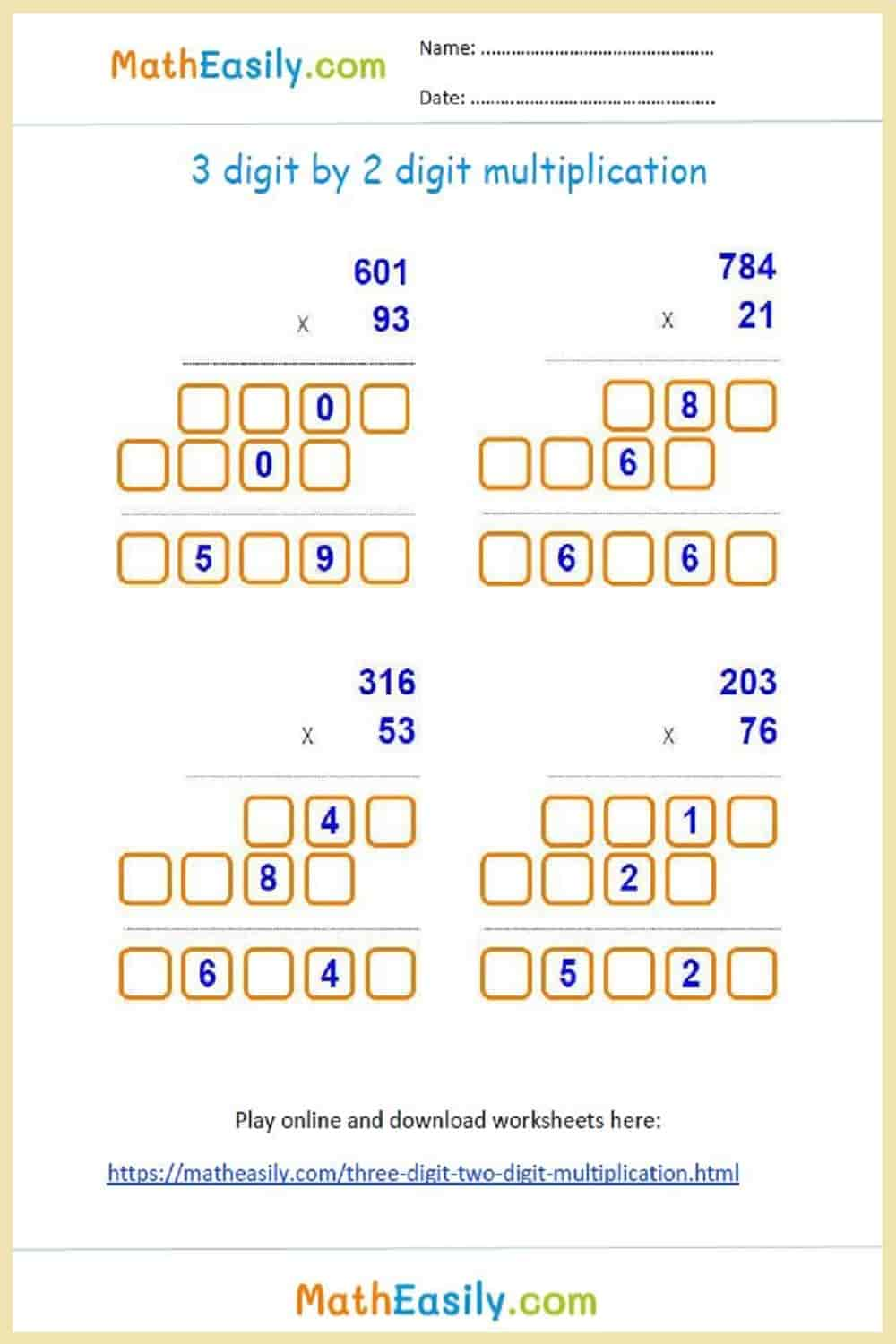### 3 digit by 3 digit

Multiply 3 digit by 3 digit numbers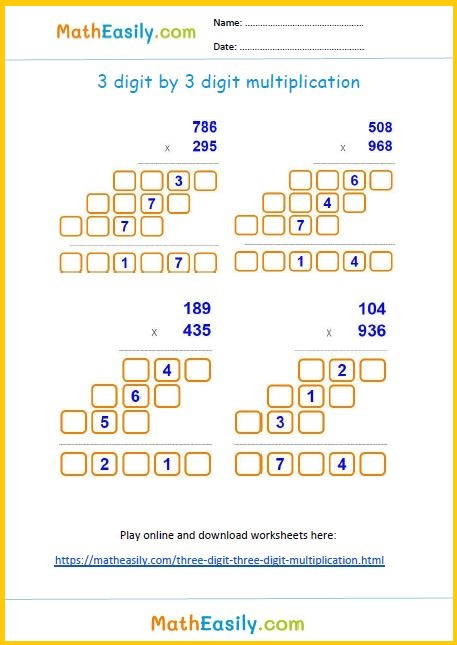### 3 digit by 3 digit

Multiply 3 digit by 3 digit numbers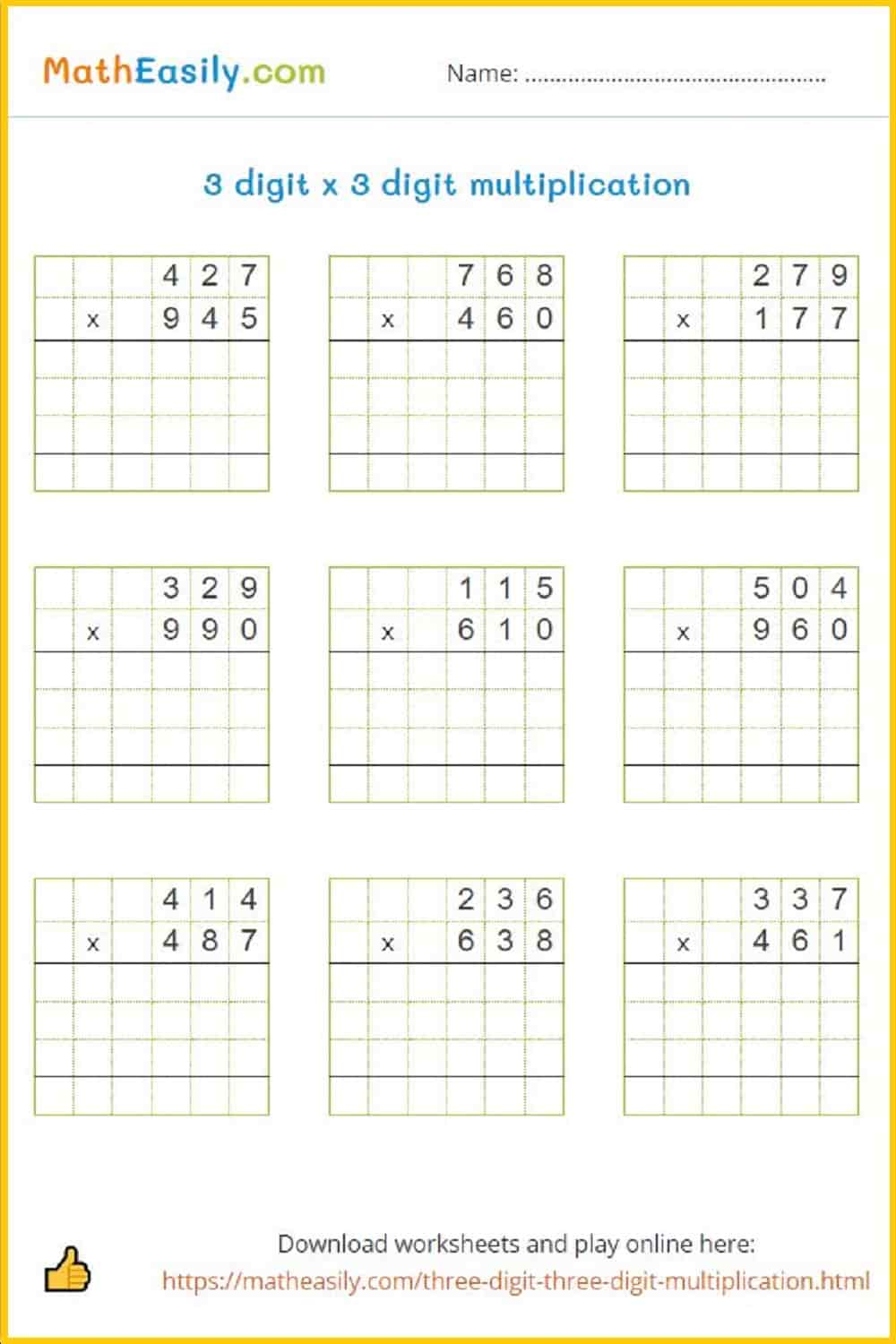### 3 digit by 3 digit

Multiply 3 digit by 3 digit numbers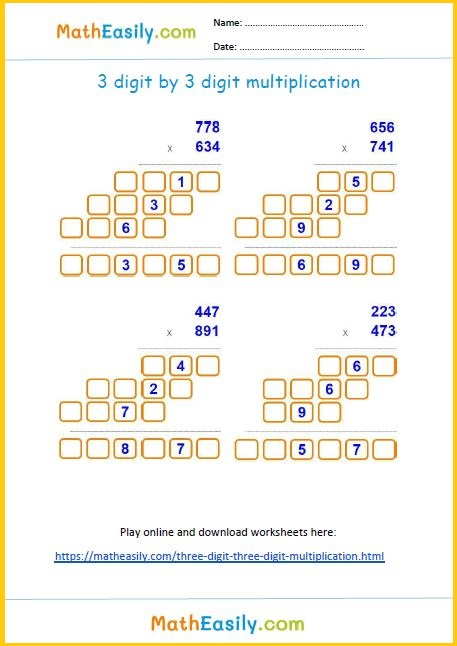## Math Worksheets for 5th Grade

Our free fifth grade math worksheets are PDF documents with the answers on the 2nd page. These math worksheets for 5th grade cover addition, subtraction, multiplication, division, fractions and much more. You may freely use any of the printable math worksheets for Grade 5 in your classroom or at home. Just click on the math worksheet picture and download the PDF.